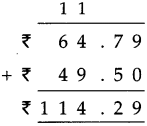# Maharashtra Board Class 5 Maths Solutions Chapter 9 Decimal Fractions Problem Set 41

Balbharti Maharashtra Board Class 5 Maths Solutions Chapter 9 Decimal Fractions Problem Set 41 Textbook Exercise Important Questions and Answers.

## Maharashtra State Board Class 5 Maths Solutions Chapter 9 Decimal Fractions Problem Set 41

Question 1.
Convert the following into decimal fractions and add them.

(1) ‘One and a half metre’ and ‘two and a half metres’
Solution: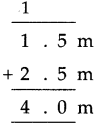(2) ‘Five and three quarter rupees’ and ‘seven and a quarter rupees’
Solution: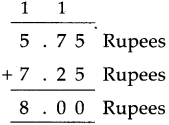(3) ‘Six and a half metres’ and ‘three and three quarter metres’.
Solution: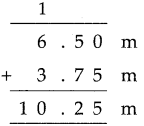Question 2.
(1) 23.4 + 87.9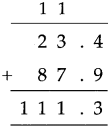(2) 35.74 + 816.6
Solution: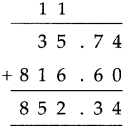(3) 6.95 + 74.88
Solution: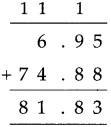(4) 41.03 + 9.98
Solution: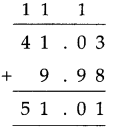Question 3.
(1) 51.4 cm + 68.5 cm
Solution: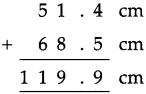(2) 94.7 m + 1738.45 m
Solution: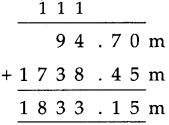(3) 5158.75 + `841.25
Solution: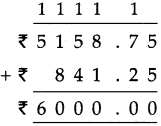Subtraction of decimal fractions

Study the subtraction of decimal fractions given below.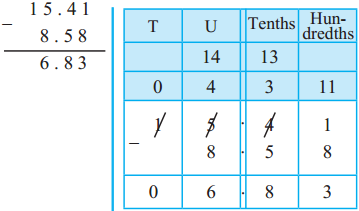8 hundredths cannot be subtracted from 1 hundredth, so 1 tenth (or 10 hundredths) from 4 tenths are borrowed. The borrowed 10 hundredths and the original one hundredth make 11 hundredths. 11 hundredths minus 8 hundredths are 3 hundredths. They are written in the hundredths place under the line. The rest of the subtraction is carried out using the same method.

Convert the following into decimal fractions and add them.

(1) ‘Fourteen and a half rupees’ and ‘Fifteen and a half rupees’.
Solution: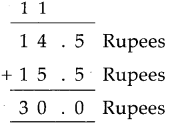(2) ‘Three quarters’ and ‘a half’
Solution: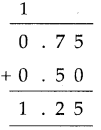(1) 37.84 + 12.16
Solution: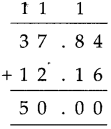(2) 328.69 + 84.84
Solution: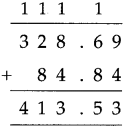Solve the following:

(1) 304.86 m + 70.94 m
Solution: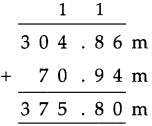(2) 79.56 cm + 19.65 cm
Solution: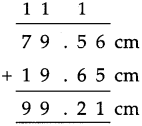(3) ₹ 64.79 + ₹  49.5
Solution: